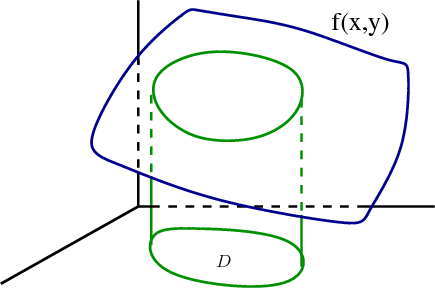# Math Insight

### Image: Double integral as volume under a surfaceThe double integral $\iint_{\dlr} f(x,y) dx\,dy$ for $f(x,y)>0$ can be interpreted as the volume under the surface $z =f(x,y)$ over the region $(x,y) \in \dlr$.

Image file: double_integral_volume_under_surface.png